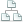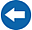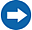Home >Tips >Excel 2016 from Scratch >Calculate Sums DSite map

# Excel 2016 from Scratch Exercise Calculate Sums D

## An Excel tutorial by Peter KalmstromIn this demo in the Excel 2016 from Scratch series, Peter Kalmstrom explains how to use the AutoSum function in different ways.

Peter uses sales of hardware from stores in four cities for each quarter as an example. He calculates the sum for each town and hardware and quater using AutoSum and formulas, and calculates total budget of all cells by using formulas.

### Exercise

If you want to try the exercise yourself, please download the Excel file Peter uses in the demo!

### Content

This is what Peter shows in the demo below:
• How to use AutoSum to calculate the sum of the values in one row.
• How to apply a formula on a whole column.
• How to use the AutoSum function in different ways on cells.
• How to copy format from the Format Painter and apply on multiple cells.
• How to select different cells and calculate the sum of the values in those cells.
Peter uses Excel 2016 for his demo, but the Excel basics are the same for earlier versions of Excel.